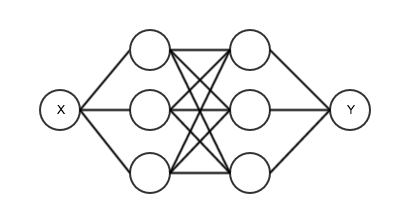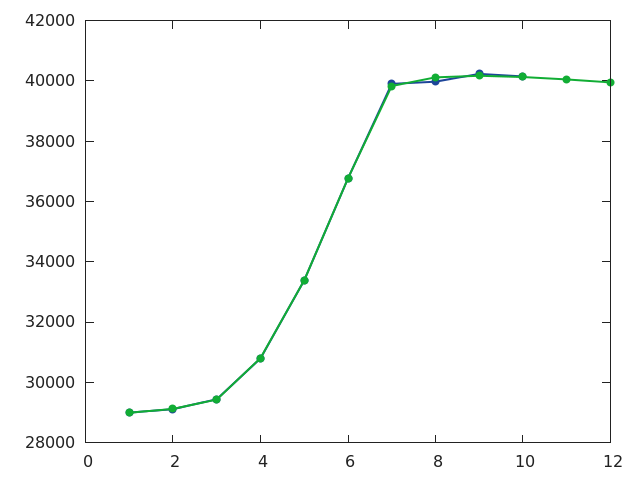``model = nn.Sequential()``

``model:add(nn.Linear(1,3))``

``model:add(nn.Sigmoid())``

``model:add(nn.Linear(3,3))``

``model:add(nn.Sigmoid())``

``model:add(nn.Linear(3,1))``

``````model = nn.Sequential()

``````model = nn.Sequential()

``require 'optim'``

``w, dl_dw = model:getParameters()``
w是model裡面所有可調引數的集合，dl_dw是每個引數對loss的偏導數。需要注意的是這裡的w和dl_dw都相當於C++裡面的“引用”，一旦你對他們進行了操作，模型裡的引數也會跟著改變。

``````feval = function(w_new)
if w ~= w_new then w:copy(w_new) end
dl_dw:zero()

price_predict = model:forward(month_train)
loss = criterion:forward(price_predict, price_train)
model:backward(month_train, criterion:backward(price_predict, price_train))
return loss, dl_dw
end``````

``````params = {
learningRate = 1e-2
}

for i=1,3000 do
optim.rprop(feval, w, params)

if i%10==0 then
gnuplot.plot({month, price}, {month_train:reshape(10), price_predict:reshape(10)})
end
end``````

``th mlp.lua````````require 'torch'
require 'nn'
require 'optim'
require 'gnuplot'

month = torch.range(1,10)
price = torch.Tensor{28993,29110,29436,30791,33384,36762,39900,39972,40230,40146}

model = nn.Sequential()
criterion = nn.MSECriterion()

month_train = month:reshape(10,1)
price_train = price:reshape(10,1)

gnuplot.figure()

w, dl_dw = model:getParameters()

feval = function(w_new)
if w ~= w_new then w:copy(w_new) end
dl_dw:zero()

price_predict = model:forward(month_train)
loss = criterion:forward(price_predict, price_train)
model:backward(month_train, criterion:backward(price_predict, price_train))
return loss, dl_dw
end

params = {
learningRate = 1e-2
}

for i=1,3000 do
optim.rprop(feval, w, params)

if i%10==0 then
gnuplot.plot({month, price}, {month_train:reshape(10), price_predict:reshape(10)})
end
end

month_predict = torch.range(1,12)
local price_predict = model:forward(month_predict:reshape(12,1))
print(price_predict)

gnuplot.pngfigure('plot.png')
gnuplot.plot({month, price}, {month_predict, price_predict})
gnuplot.plotflush()``````